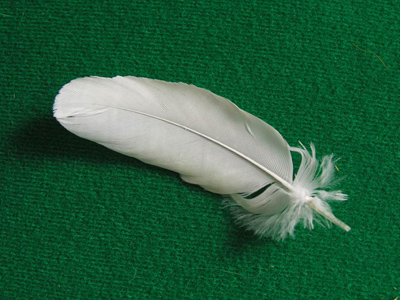A tonne of feathers weighs the same as a tonne of bricks!

# Measures 3 (Medium)

Now that you have made it to the third of our Eleven Plus maths quizzes on Measures, you should know how imperial and metric units of measurement compare. In previous quizzes, we gave you a hand by telling you how to convert one to the other. Now it’s time to see how much you can remember!

It is very easy to change miles into kilometres if the formula is there in front of you, but can you do it all on your own? If not, don’t worry. Just make sure you read the helpful comments after each question. They will explain anything you need to know.

If you don’t get all the questions right first time, why not try the quiz again? Repetition is the best way to learn. And, if you haven’t already played our previous quizzes on measures, then I suggest you do before you try this one. They’ll help you to get the measure of measures!

1.
Which one of the following statements is false?
There are 1,000 kg in a tonne
There are 14 pounds in a stone
There are 1,000 centilitres in a litre
There are 1,760 yards in a mile
There are 1,000 millilitres in a litre, but only 100 centilitres. ‘Milli’ means a thousandth, ‘centi’ means a hundredth
2.
Which of the following lengths is the longest: 5 km, 4 miles, 4,800 metres or 1,760 yards?
5 km
1,760 yards
4 miles
4,800 metres
5 km = 5,000 metres. 1 mile = 1,760 yards. To convert miles to km, a quick rule is to divide by 5 then times by 8: 4 ÷ 5 = 0.8, 0.8 x 8 = 6.4 km, so 4 miles is the longest distance
3.
How many millimetres are there in 1.83 metres?
1,830 mm
183 mm
18,300 mm
183,000 mm
To convert metres to millimetres simply multiply by 1,000:
1.83 x 1,000 = 1,830
4.
Which of the following is the largest volume: 1 pint, 1 litre, 2,500 ml or 1 gallon?
1 pint
1 litre
2,500 ml
1 gallon
1 pint = 0.568 litres, 2,500 ml = 2.5 litres, 1 gallon = 8 pints or 0.568 x 8 = 4.544 litres, so a gallon is the largest volume
5.
A Marathon is a race over a distance of 42,195 metres. How many km is that?
4.2195 km
42.195 km
421.95 km
4,219.5 km
1 km = 1,000 m, so to convert metres to km, just divide by 1,000: 42,195 ÷ 1,000 = 42.195
6.
What is 568 ml the same as?
A quart
Two pints
Half a litre
A pint
568 ml = 0.568 l = 1 pint. Learn this if you can
7.
Which of the following is the same as 20,000 cm2?
1 yard3
1 m3
2 yard2
2 m2
1 m2 = 10,000 cm2: 100 x 100 = 10,000
8.
The area of a square is 144 cm2. What is the length of one of its sides?
3.5 cm
12 cm
24 cm
36 cm
The square root (√) of 144 is 12: 12 x 12 = 144. You’d do well to remember the squares of as many numbers as you can
9.
Which is bigger: a metric ton (tonne) or an Imperial ton?
A ton
A tonne
They are both the same
It depends on what is being weighed
1 tonne = 1,000 kg, 1 ton = 1,016 kg.
It doesn’t matter what you are weighing: a tonne of feathers weighs the same as a tonne of bricks!
10.
A cube has a volume of 64 cm3. How wide is the cube?
2 cm wide
4 cm wide
6 cm wide
8 cm wide
Volume = height × width × length, so a cubed number is that number multiplied with itself twice: 4 x 4 x 4 = 64. It’s a good idea to learn the cubes of as many numbers as you can
Author:  Frank Evans